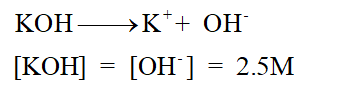# Hi,Q: Determine [OH- ] in each base solution.  If the acid is weak, indicate the value that [OH- ] is less than,a) 2.5 M KOHb) 0.225 M Ba(OH)2c) 1.8M C5H5N=:-)

Question
6 views

Hi,

Q:

Determine [OH- ] in each base solution.  If the acid is weak, indicate the value that [OH- ] is less than,

a) 2.5 M KOH

b) 0.225 M Ba(OH)2

c) 1.8M C5H5N

=:-)

check_circle

Step 1

The Bronsted-Lowry acid-base theory was purposed by Bronsted and Lowery is called Bronsted-Lowry acid-base theory. It states that acid can give H+ ions whereas a base can accept the  ion in its solution. Hence this theory is entirely based on the presence of H+ ion in the given substance.  It purposed the concept of conjugate acid-base pair. A Bronsted acid gives H+  ion to form conjugate base whereas a Bronsted base accepts H+ ion to form its conjugate acid.

Step 2

The equilibrium constant for acid dissociation is denoted as Ka. It represents the ratio of the equilibrium concentration of product and reactant molecule. Similarly the equilibrium constant for base dissociation is denoted as Kb. It represents the ratio of the equilibrium concentration of product and reactant molecule.

Step 3

Part (a)

KOH is a strong base and since it is a monoacidic base therefore the concentration of KOH must be the concentration of OH- ions....

### Want to see the full answer?

See Solution

#### Want to see this answer and more?

Solutions are written by subject experts who are available 24/7. Questions are typically answered within 1 hour.*

See Solution
*Response times may vary by subject and question.
Tagged in